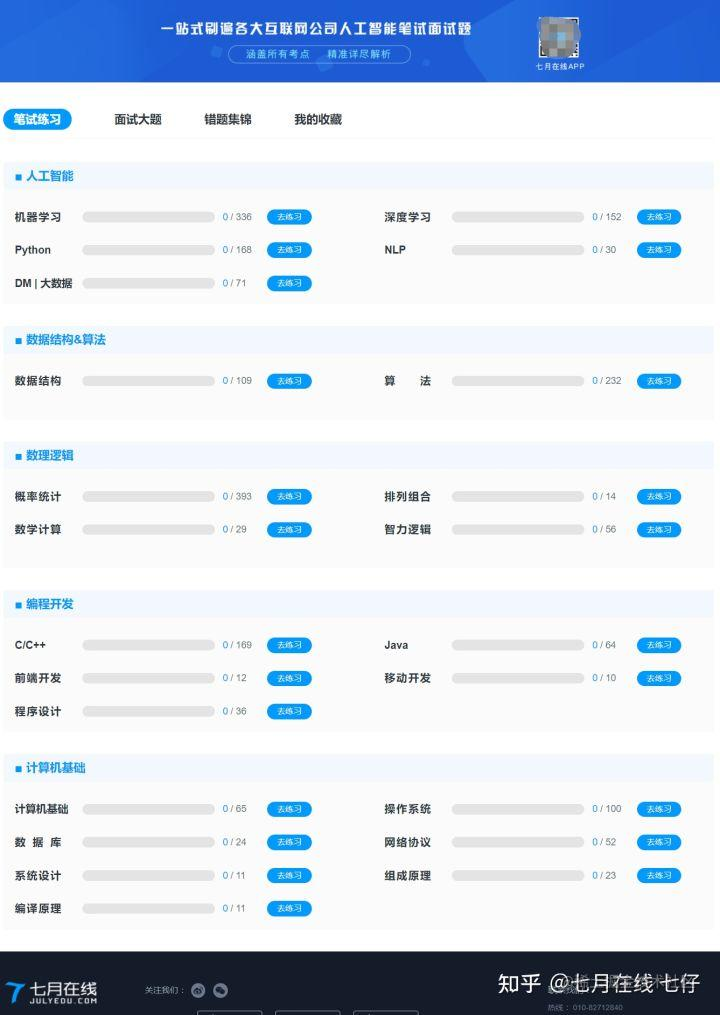# 2021年6月底--7月3日，拼多多搜索广告算法暑假实习面试题2道

## 1. 算法题：leetcode 687. 最长同值路径

``````class Solution(object):
def longestUnivaluePath(self, root):
self.ans = 0

def arrow_length(node):
if not node: return 0
left_length = arrow_length(node.left)
right_length = arrow_length(node.right)
left_arrow = right_arrow = 0
if node.left and node.left.val == node.val:
left_arrow = left_length + 1
if node.right and node.right.val == node.val:
right_arrow = right_length + 1
self.ans = max(self.ans, left_arrow + right_arrow)
return max(left_arrow, right_arrow)

arrow_length(root)
return self.an## 2. 算法题：leetcode 322. 零钱兑换

dp[j]代表含义：填满容量为j的背包最少需要多少硬币

``````class Solution:
def coinChange(self, coins: List[int], amount: int) -> int:
dp =  +  * amount
for coin in coins:
for j in range(coin, amount + 1):
dp[j] = min(dp[j], dp[j - coin] + 1)
return dp[-1] if dp[-1] != 10001 else -1